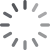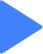• 什么是随堂模式？

随堂模式课程一般为每学期一轮次，课程每周更新，作业、考试有截止时间，由课程提供方老师、助教指导，课程完结，成绩由老师确认后，统一发放证书。

• 什么是自主模式？

自主模式课程常年开放加入，课件全部开放，作业、考试无截止时间，有学堂在线招募选拔的助教指导，考核通过即可自动获得证书。展开

课程章节

MATLAB课程学习 MATLAB Learning Content
MATLAB介绍 MATLAB Intro
MATLAB向量 MATLAB Vectors
MATLAB矩阵 MATLAB Matrices
MATLAB画图 MATLAB Plotting
MATLAB求解器 MATLAB Solver

授课教师

•J. Kim Vandiver麻省理工学院 本科生研究系 系主任

J. Kim Vandiver 教授是麻省理工学院本科生研究系主任以及埃杰顿中心主任。他于 1992 年在麻省理工学院创建了埃杰顿中心，以期为麻省理工学院的学生追寻各自创造性项目提供资源支持。该中心运营着3家学生店，并为很多学生团队提供资助，其中包括太阳能电动车团队、大学生方程式赛车团队和海洋机器人团队。他是机械和海洋工程学教授